• ## python生成二维矩阵的方法

万次阅读 多人点赞 2018-07-24 16:46:32
1、直接用列表生成m行n列的矩阵 m,n = map(int,input().split()) matrix = [*(m)]*(n) 输出为： 这种方式生成矩阵存在一定的问题，比如，无法给特定位置的元素赋值，例如： matrix = 9 输出为：...
欢迎关注公众号——《数据三分钟》

一线大厂的师兄师姐结合自己的工作实践，将数据知识浅显道来，每天三分钟，助你成为数据达人。还有面试指导和内推机会。1、直接用列表生成m行n列的矩阵

m,n = map(int,input().split())
matrix = [*(m)]*(n)

输出为：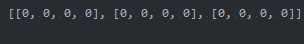这种方式生成的矩阵存在一定的问题，比如，无法给特定位置的元素赋值，例如：

matrix = 9

输出为：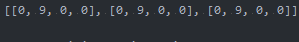可见，第二列的元素全部被赋值为9了

2、采用numpy生成想要维度的矩阵

import numpy as np
x,y = map(int,input().split())
a = np.ones((x+1,y+1))

输出为：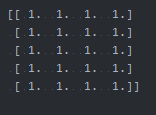上面的输出特别漂亮，一个完美的矩阵形式输出，下面我们试一下修改特定位置的元素值。

import numpy as np
x,y = map(int,input().split())
a = np.ones((x+1,y+1))
a = 9
print(a)

输出为：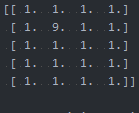可见，我们成功修改了第二行第二列的元素值，因此通过numpy生成的矩阵更具有可操作性。比如下面的操作：

import numpy as np
x,y = map(int,input().split())
a = np.ones((x+1,y+1))
for i in range(1,x+1):
for j in range(1,y+1):
a[i][j] = a[i-1][j] + a[i][j-1]
print(a)

输出为：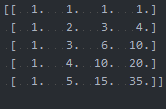展开全文• Python 输出二维矩阵固定元素的坐标 import numpy as np y=np.array([[1,0,1,1],[1,0,1,2],[1,0,1,1],[1,0,1,1]])#定义矩阵 print(np.argwhere(y==1))#输出一维列表y=1时的坐标! [在这里插入图片描述]...
Python 输出二维矩阵固定元素的坐标
import numpy as np
y=np.array([[1,0,1,1],[1,0,1,2],[1,0,1,1],[1,0,1,1]])#定义矩阵
print(np.argwhere(y==1))#输出一维列表y=1时的坐标!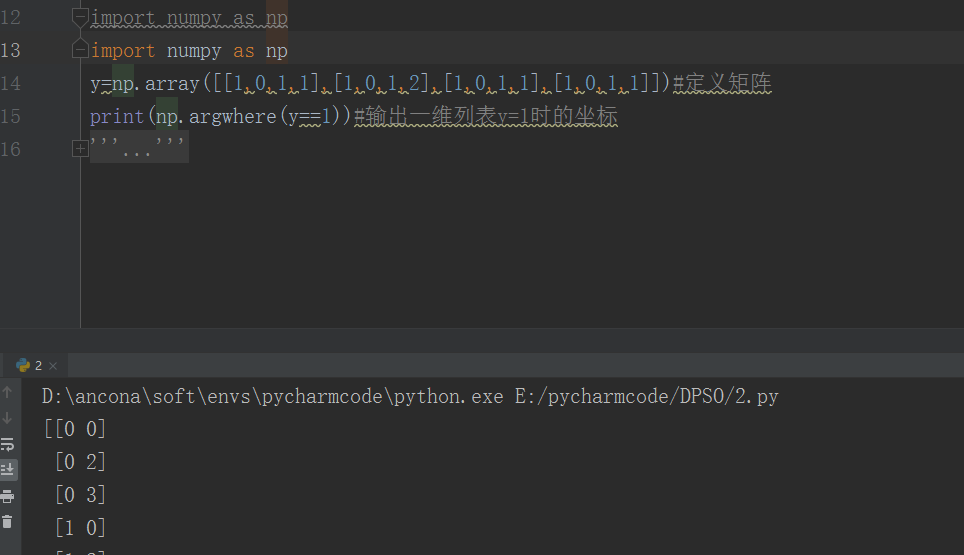展开全文• Python二维矩阵沿主对角线（次对角线）翻转变换代码实现 目录Python二维矩阵沿主对角线（次对角线）翻转变换代码实现1. 原始数据以及图示2. 主对角线翻转及图示3. 次对角线翻转结果4. 完整代码，如有疑问，欢迎...
Python对二维数组（矩阵）沿主对角线（次对角线）翻转变换代码实现
目录Python对二维数组（矩阵）沿主对角线（次对角线）翻转变换代码实现1. 原始数据以及图示2. 主对角线翻转及图示3. 次对角线（副对角线）翻转结果4. 完整代码，如有疑问，欢迎评论区交流
1. 原始数据以及图示
为了展现代码的效果，这里选取了一个3*3的数组，分别是0-8的值，便于区分。为了直观展示数据，这里使用了热力图用颜色区分数值大小。关于如何绘制，参见：https://blog.csdn.net/zzu_Flyer/article/details/109309082
原始矩阵如下:2. 主对角线翻转及图示3. 次对角线（副对角线）翻转结果4. 完整代码，如有疑问，欢迎评论区交流
import seaborn as sns
import numpy as np
import matplotlib.pyplot as plt
# 显示中文字体
from pylab import *
mpl.rcParams['font.sans-serif']=['SimHei']
# n数组维度
n = 3
my_array = np.zeros((n, n))
# 随机生成原始数组
s = 0
for i in np.arange(n):
for j in np.arange(n):
print(i, j)
my_array[i, j] = s
s = s + 1
print(my_array)

# 沿主对角线数组翻转
def fanzhuan_zhu(wind_array, nx):
for i in range(nx):
# print('i', i)
for j in range(i, nx - i):
print('--',i, j)
tmp = wind_array[i, j]
wind_array[i, j] = wind_array[j, i]
wind_array[j, i] = tmp
j = j + 1
i = i + 1
return wind_array

# 沿副对角线数组翻转
def fanzhuan_fu(wind_array, nx):
for i in range(nx - 1):
for j in range(nx - 1 - i):
print(i, j)
tmp = wind_array[i, j]
wind_array[i, j] = wind_array[nx - j - 1, nx - i - 1]
wind_array[nx - j - 1, nx - i - 1] = tmp
j = j + 1
i = i + 1
return wind_array

# 绘制热力图
def show(wind_array, title):
# 绘制热力图
h = sns.heatmap(
data=wind_array,  # 指定绘图数据
cmap='YlGnBu',  # 指定填充色'PuBuGn'，，jet
# center=1,
linewidths=.1,  # 设置每个单元格边框的宽度
annot=True,  # 显示数值
cbar=True,
fmt='.1f',# 以科学计算法显示数据
)
plt.title(title, fontsize=15)
plt.tight_layout()

# 显示图形
plt.show()

show(my_array,'原始数组')
zhu = fanzhuan_zhu(my_array, n)
print('主对角线翻转',zhu)

show(zhu, '主对角线翻转后')

fu = fanzhuan_zhu(my_array, n)
print('副对角线翻转', fu)
show(fu, '副对角线翻转后')




展开全文数组
• 因为做项目中间有一个很小的环节需要这个功能，所以就...usr/bin/env python #encoding:utf-8 ''' __Author__:沂水寒城 ''' def get_max_value(martix): ''' 得到矩阵中每一列最大的值 ''' res_list=[] for j
    因为做项目中间有一个很小的环节需要这个功能，所以就写了一个简单的小函数，下面是具体实现：

#!usr/bin/env python
#encoding:utf-8

'''
__Author__:沂水寒城
'''

def get_max_value(martix):
'''
得到矩阵中每一列最大的值
'''
res_list=[]
for j in range(len(martix)):
one_list=[]
for i in range(len(martix)):
one_list.append(int(martix[i][j]))
res_list.append(str(max(one_list)))
return res_list

if __name__ == '__main__':
martix=[['1','2','3'],['3','5','0'],['5','6','2']]
print get_max_value(martix)
结果如下：

['5', '6', '3']`
展开全文• 今天，遇上一个需求就是根据数据生成图像，当然不仅仅是这么简单，但是突然觉得很好玩，就简单实验了一下，随机的生成二维的数据矩阵，然后使用这个随机矩阵的数据来生成随机的图像，仅仅是好玩，下面是具体的实现：...
• 最简单易懂的方法，这种逻辑不仅适用于 python，也适用于任何变成语言。 row = 5 col = 8 matrix = [] for i in range(row): m = [] for j in range(col): m.append(False) matrix.append(m) ...
• 简述 网上方法有很多种。 这里就先记录下，一般人都想不到的一种来试试看~ ...关于这个解释，是用到我之前写过的一篇快速生成希尔伯特...一行代码生成希尔伯特矩阵Python矩阵迅速扩展操作 点击就可以访问了~
• numpy中meshgrid函数接收两个一维数组，并产生两个二维矩阵  import numpy as np points=np.arange(-5,5,0.01) #生成1000个间隔相等的点 xs,ys=np.meshgrid(points,points) ys import matplotlib.pyplot as ...
• 生成二维数组 word = np.zeros([2, 4])#生成一个二维四列的矩阵 word[0, 0] = 0 #赋值 word[0, 1] = 1 word[0, 2] = 2 word[0, 3] = 3 word[1, 0] = 4 word[1, 1] = 5 word[1, 2] = 6 word[1, 3] = 7 print(word) ...numpy
• 简单使用min、max函数来得到二维数据矩阵中的最大最小值，很简单，这是因为工作需要用到一个东西所以先简单来写了一下： #!usr/bin/env python #encoding:utf-8 ''' __Author__:沂水寒城 功能：找出来随机生成矩阵...
• 用列表解析可以快捷地生成二维数组，代码如下 n,m=map(int,input().split()) graph=[[0 for j in range(n)] for i in range(m)] print(graph)
• 随机生成一个样本容量为1000的二维正态分布样本,给出相应分布协方差矩阵的估计,比较估计值和真实值之间的差,分析提高估计精度的方法.
• python 生成对称矩阵Prerequisite: 先决条件： Defining Matrix using Numpy 使用Numpy定义矩阵 Transpose Matrix 转置矩阵 Here, we will learn how to create a symmetric matrix using a non-symmetric matrix?...机器学习 numpy tensorflow 人工智能
• data_count=np.zeros((21, 6), dtype=int) 参考链接
• 生成numpy矩阵的几个相关函数： numpy.array() numpy.zeros() numpy.ones() numpy.eye() 串联生成numpy矩阵的几个相关函数： numpy.array() numpy.row_stack() numpy.column_stack() numpy.reshape()numpy
• 主要介绍了Python使用min、max函数查找二维数据矩阵中最小、最大值的方法,涉及Python矩阵生成、遍历、查找等相关操作技巧,需要的朋友可以参考下
• 生成随机数 使用 random 模块 random.random() 用于随机生成一个0到1的浮点数 random.randint(start,stop) 随机生成[start,stop]区间内的整数 代码示例： import random print (random.random()) print(random....
• 比如在实现逻辑回归和线性回归等算法时，需要先初始化一个权值矩阵，也就是要自动生成一个固定大小且各值全部相等的二维矩阵，这时候就可以用numpy来实现了。 下面的代码就是生成一个x行y列的全为0的矩阵。 import...numpy 数据分析
• 前言：今天为大家带来的内容是，详解：python numpy矩阵的创建与数据类型！...使用python中的方法构造矩阵- 生成维矩阵# 使用python自带的range（）方法生成一个矩阵a = list(range(100))#ran...
• 正确的方式 通过嵌套for循环，一个个添加0 n = [] for i in range(2): n.append([]) for j in range(3): n[i].append(0) n = 2 print(n) 输出[[2, 0, 0], [0, 0, 0]] ...输出[[2, 0, 0...列表
• Python 创建二维列表list 2018年12月26日 10:08:49 蔷薇的天空 阅读数 2475 Python 创建二维列表list 1.正确使用方式： 2.误用方式： 原因：matrix = [array] * 3操作中，只是创建3个指向array的引用，所以一旦array...
• python中有两个模块可以生成随机数，该博客以的numpy模块为例进行生成随机数。（因为矩阵生成大量的随机数据，故推荐使用numpy模块生成随机数） 生成随机数（以矩阵为例） # 生成随机矩阵 import numpy as np #　...随机数
• 1、绘图横纵坐标轴倒转问题 今天使用了python绘制三维图像的二维切片，把Y固定为某一个值绘制X-Z切片，但是绘制出来的结果图发现X轴和Z轴发生了倒转，...如果生成一个二维矩阵，我们会习惯性地把第一个数默认为x，第二
• ------------------在python中导出矩阵至matlab------------------------------ 一、通过文本文件 如果矩阵是mxn的。 那么可以用 : np.savetxt('dev_ivector.csv', dev_ivector, delimiter = ',') 对应matlab...
• python中的numpy.random.randint(a,b)用于生成一个指定范围内的c行d列的整数矩阵。其中参数a是下限，参数b是上限，生成的随机数矩阵n: a <= n <= b。前提是必须import numpy，如果直接import random后调用...txt存储
• 这里介绍一下高斯分布蒙版的特性，并且用Python实现。 高斯分布的蒙版，简单来说，就是一个从中心扩散的亮度分布图，如下所示： 亮度的范围从 1 到 0, 从中心到边缘逐渐减弱，中心的亮度值最高为1，边缘...图像处理
• 利用python生成一个矩阵矩阵需要满足以下要求： 1.矩阵的每个元素都是数字 2.矩阵任两行各不相同 3.矩阵的每一列的所有元素均要小于某个值（每一列约束不同，比如第一列需要小于8，第列小于10...） 输入......

# python生成二维矩阵python 订阅